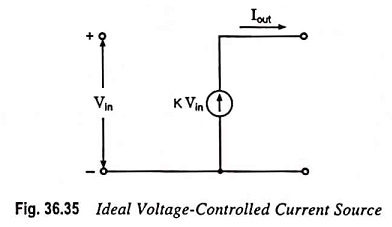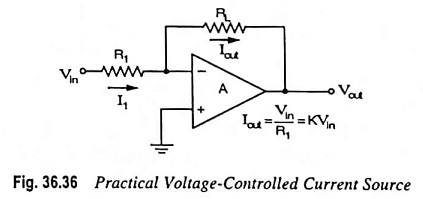## Voltage Controlled Current Source (VCCS) Circuit:

An ideal form of a current source, whose output current Iout is controlled by an input voltage is shown in Fig. 36.35. Here, the output current Iout is dependent on input voltage Vin.A practical circuit can be built, as shown in Fig. 36.36, with the output or load current through load resistor RL controlled by the input voltage Vin. The current through load resistor RL can be seen to be Iout = Vin/R1 = KVin.Since, the output resistance is (ideally) zero, there is no voltage division at the output and the output voltage is independent of load.

Scroll to Top